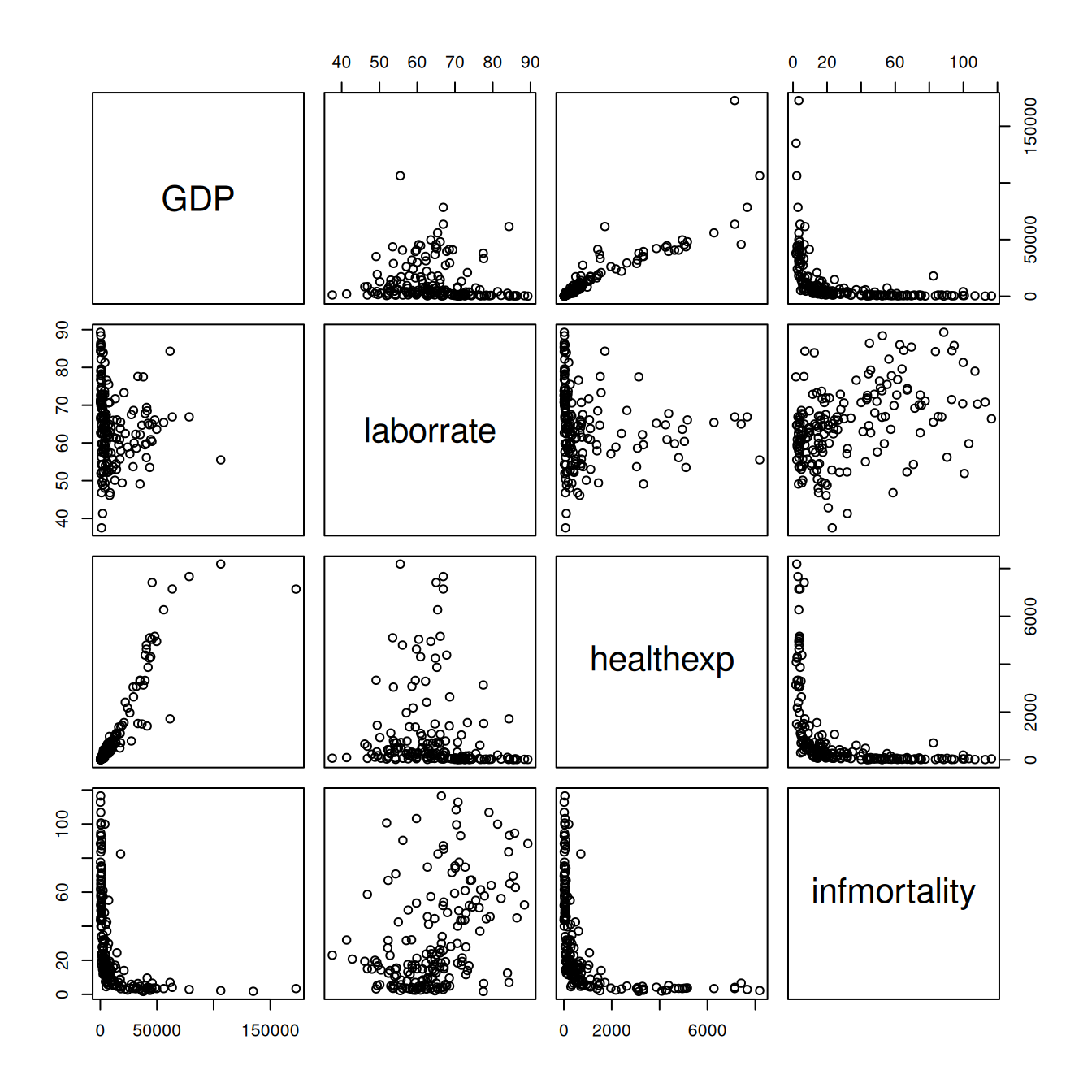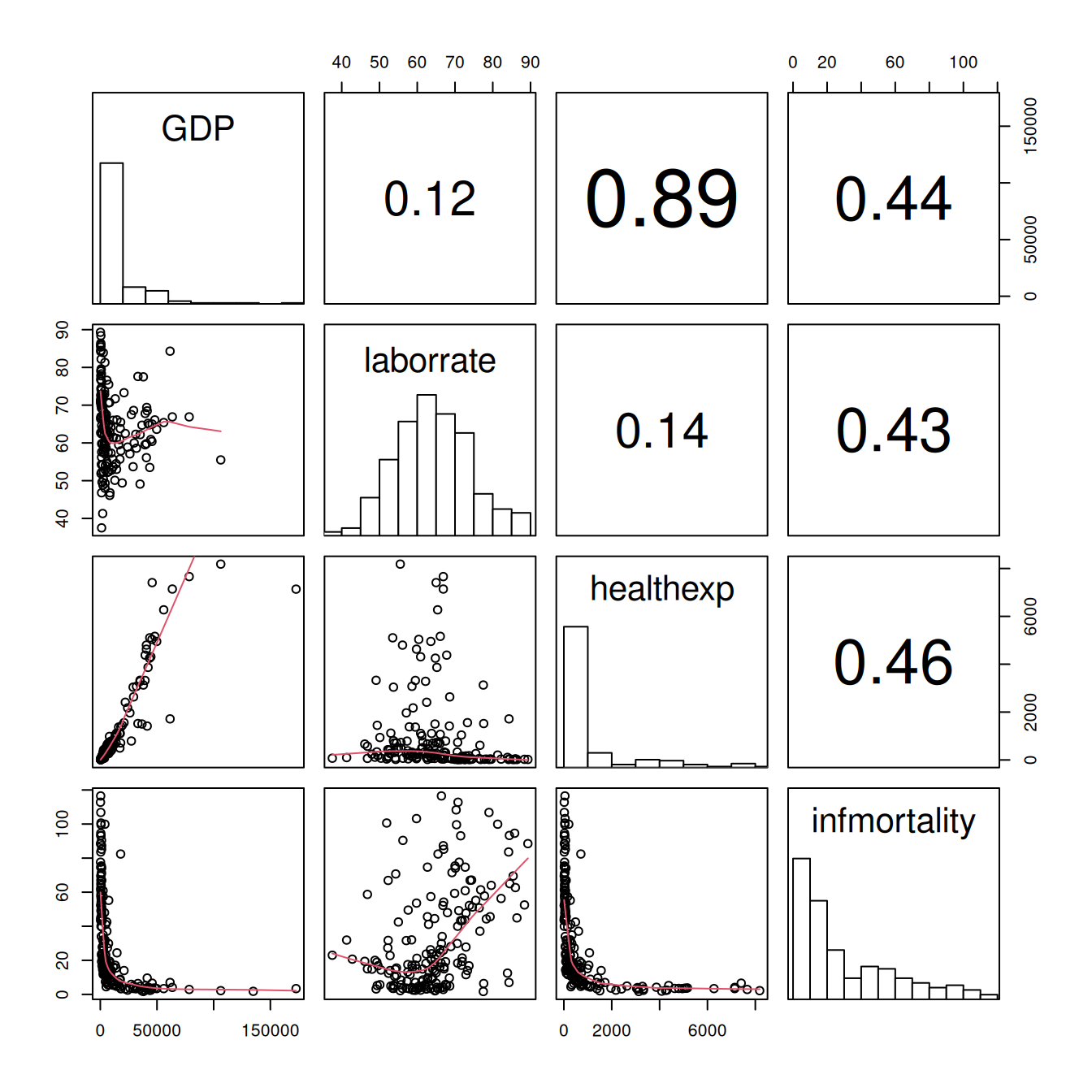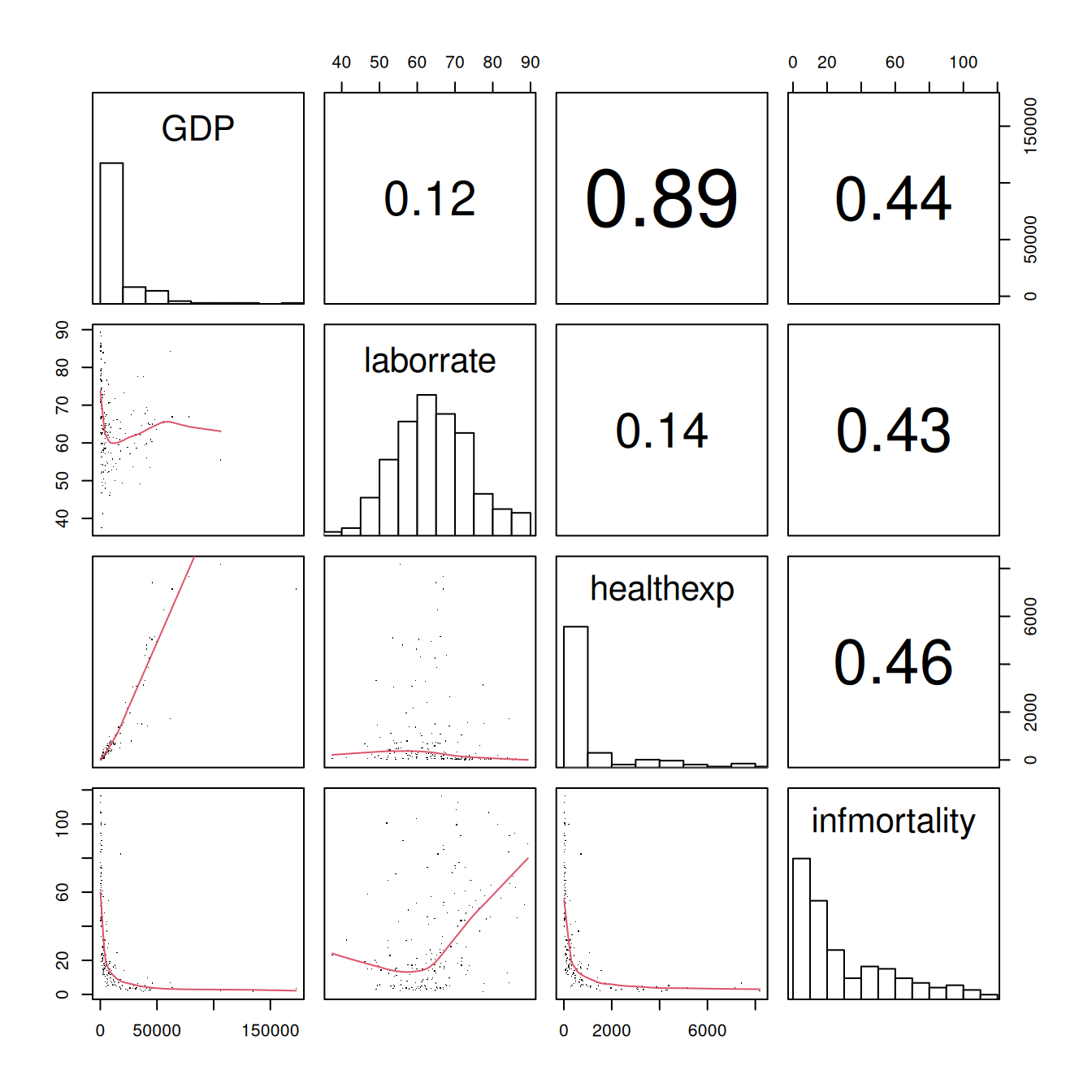## 5.13 Making a Scatter Plot Matrix

### 5.13.1 Problem

You want to make a scatter plot matrix.

### 5.13.2 Solution

A scatter plot matrix is an excellent way of visualizing the pairwise relationships among several variables. To make one, use the `pairs()` function from R’s base graphics.

For this example, we’ll use a subset of the `countries` data. We’ll pull out the data for the year 2009, and keep only the columns that are relevant:

``````library(gcookbook) # Load gcookbook for the countries data set

c2009 <- countries %>%
filter(Year == 2009) %>%
select(Name, GDP, laborrate, healthexp, infmortality)

c2009
#>            Name       GDP laborrate healthexp infmortality
#> 1   Afghanistan        NA      59.8  50.88597        103.2
#> 2       Albania 3772.6047      59.5 264.60406         17.2
#> 3       Algeria 4022.1989      58.5 267.94653         32.0
#>  ...<210 more rows>...
#> 214 Yemen, Rep. 1130.1833      46.8  64.00204         58.7
#> 215      Zambia 1006.3882      69.2  47.05637         71.5
#> 216    Zimbabwe  467.8534      66.8        NA         52.2``````

To make the scatter plot matrix (Figure 5.38), we’ll use all of the variables except for `Name`, since making a scatter plot matrix using the names of the countries wouldn’t make sense and would produce strange-looking results:

``````c2009_num <- select(c2009, -Name)
pairs(c2009_num)``````Figure 5.38: A scatter plot matrix

### 5.13.3 Discussion

You can also use customized functions for the panels. To show the correlation coefficient of each pair of variables instead of a scatter plot, we’ll define the function `panel.cor`. This will also show higher correlations in a larger font. Don’t worry about the details for now – just paste this code into your R session or script:

``````panel.cor <- function(x, y, digits = 2, prefix = "", cex.cor, ...) {
usr <- par("usr")
on.exit(par(usr))
par(usr = c(0, 1, 0, 1))
r <- abs(cor(x, y, use = "complete.obs"))
txt <- format(c(r, 0.123456789), digits = digits)
txt <- paste(prefix, txt, sep = "")
if (missing(cex.cor)) cex.cor <- 0.8/strwidth(txt)
text(0.5, 0.5, txt, cex =  cex.cor * (1 + r) / 2)
}``````

To show histograms of each variable along the diagonal, we’ll define `panel.hist`:

``````panel.hist <- function(x, ...) {
usr <- par("usr")
on.exit(par(usr))
par(usr = c(usr[1:2], 0, 1.5) )
h <- hist(x, plot = FALSE)
breaks <- h\$breaks
nB <- length(breaks)
y <- h\$counts
y <- y/max(y)
rect(breaks[-nB], 0, breaks[-1], y, col = "white", ...)
}``````

Both of these panel functions are taken from the `pairs()` help page, so if it’s more convenient, you can simply open that help page, then copy and paste. The last line of this version of the `panel.cor` function is slightly modified, however, so that the changes in font size aren’t as extreme as with the original.

Now that we’ve defined these functions we can use them for our scatter plot matrix, by telling `pairs()` to use `panel.cor` for the upper panels and `panel.hist` for the diagonal panels.

We’ll also throw in one more thing: `panel.smooth` for the lower panels, which makes a scatter plot and adds a LOWESS smoothed line, as shown in Figure 5.39. (LOWESS is slightly different from LOESS, which we saw in Recipe 5.6, but the differences aren’t important for this sort of rough exploratory visualization):

``````pairs(
c2009_num,
upper.panel = panel.cor,
diag.panel  = panel.hist,
lower.panel = panel.smooth
)
#> Warning in par(usr): argument 1 does not name a graphical parameter

#> Warning in par(usr): argument 1 does not name a graphical parameter

#> Warning in par(usr): argument 1 does not name a graphical parameter

#> Warning in par(usr): argument 1 does not name a graphical parameter

#> Warning in par(usr): argument 1 does not name a graphical parameter

#> Warning in par(usr): argument 1 does not name a graphical parameter

#> Warning in par(usr): argument 1 does not name a graphical parameter

#> Warning in par(usr): argument 1 does not name a graphical parameter

#> Warning in par(usr): argument 1 does not name a graphical parameter

#> Warning in par(usr): argument 1 does not name a graphical parameter``````Figure 5.39: Scatter plot with correlations in the upper triangle, smoothing lines in the lower triangle, and histograms on the diagonal

It may be more desirable to use linear regression lines instead of LOWESS lines. The `panel.lm()` function will do the trick (unlike the previous panel functions, this one isn’t in the pairs help page):

``````panel.lm <- function (x, y, col = par("col"), bg = NA, pch = par("pch"),
cex = 1, col.smooth = "black", ...) {
points(x, y, pch = pch, col = col, bg = bg, cex = cex)
abline(stats::lm(y ~ x),  col = col.smooth, ...)
}``````

This time the default line color is black instead of red, though you can change it here (and with `panel.smooth`) by setting `col.smooth` when you call `pairs()`.

We’ll also use small points in the visualization, so that we can distinguish them a bit better (Figure 5.40). This is done by setting `pch = "."`:

``````pairs(
c2009_num,
upper.panel = panel.cor,
diag.panel  = panel.hist,
lower.panel = panel.smooth,
pch = "."
)
#> Warning in par(usr): argument 1 does not name a graphical parameter

#> Warning in par(usr): argument 1 does not name a graphical parameter

#> Warning in par(usr): argument 1 does not name a graphical parameter

#> Warning in par(usr): argument 1 does not name a graphical parameter

#> Warning in par(usr): argument 1 does not name a graphical parameter

#> Warning in par(usr): argument 1 does not name a graphical parameter

#> Warning in par(usr): argument 1 does not name a graphical parameter

#> Warning in par(usr): argument 1 does not name a graphical parameter

#> Warning in par(usr): argument 1 does not name a graphical parameter

#> Warning in par(usr): argument 1 does not name a graphical parameter``````Figure 5.40: Scatter plot matrix with smaller points and linear fit lines

The size of the points can also be controlled using the `cex` parameter. The default value for `cex` is 1; make it smaller for smaller points and larger for larger points. Values below .5 might not render properly with PDF output.

Other packages like `GGally` have been developed as extensions to ggplot to fill in this gap. The `ggpairs()` function from the `GGally` package makes scatter plot matrices, for example.